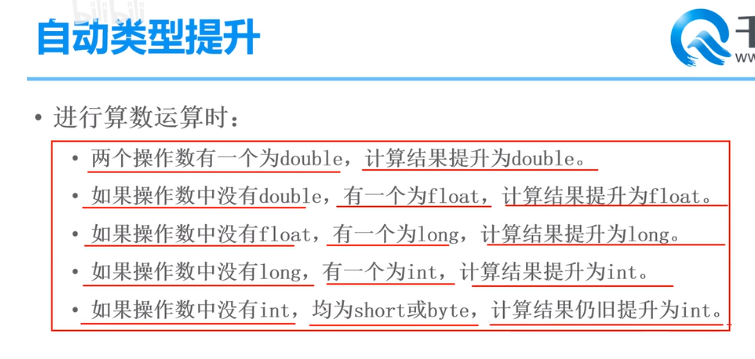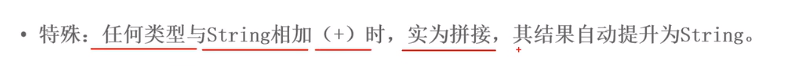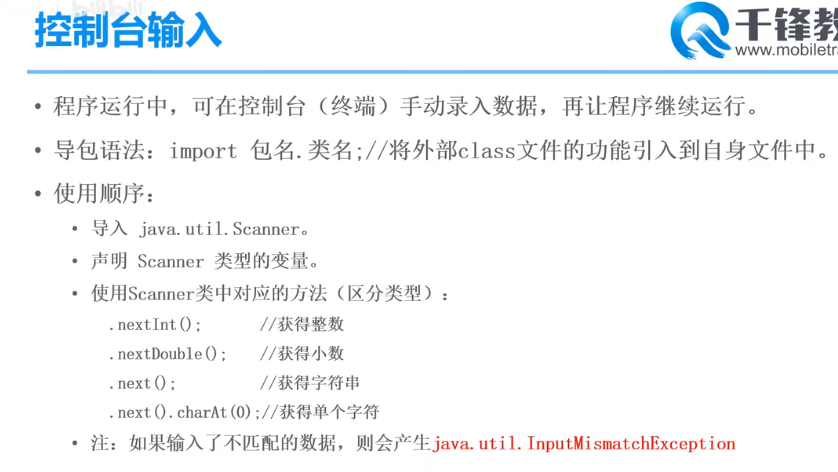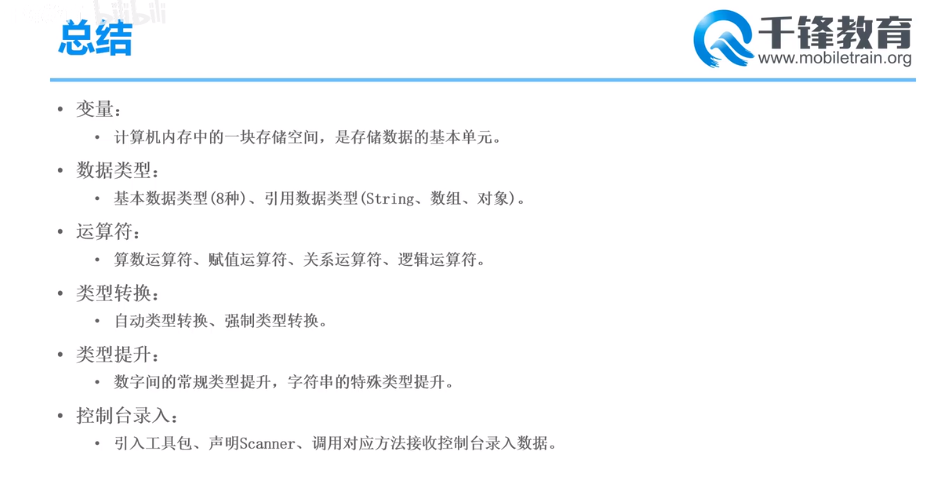# java学习04---自动类型提升/控制台输入```public class TestTypeRaise{
public static void main(String[] args) {

//自动类型提升

// 两个操作数有一个为double,返回的结果类型为double
double d1 = 10.0;
int i1 = 5;
double d2 = d1 + i1;

// 两个操作数中没有double，有一个为float,返回的结果类型为float
float f1 = 5.0F;
short s1 = 20;
float f2 = f1 + s1;

// 两个操作数中没有float，有一个为long,返回的结果类型为long
long l1 = 100;
byte b1 = 50;
long l2 = l1 + b1;

// 两个操作数中没有long，有一个为int,返回的结果类型为int
int i3 = 123;
short s3 = 456;
int i4 = i3 + s3;

// 两个操作数中没有int，都为short或byte,返回的结果类型为int

}
}
``````// 特殊：任何类型和字符串String相加（+）时，实际为和字符串进行拼接，返回的结果类型都是String
String str = "Hello";
int i5 = 123;
String str2 = str + i5;
System.out.println(str2);  // Hello123

int javaScore = 100;
System.out.println("java的成绩是：" + javaScore);
``````//package必须在源文件首行

//1.引用外部文件  jdk1.8.0_281\jre\lib\rt.jar中的文件
import java.util.Scanner;

public class TestScanner{

public static void main(String[] args) {

// 2.声明Scanner类型的变量
Scanner input = new Scanner(System.in);

System.out.println("请输入一个整数");

// 3.使用Scanner类中对应的方法(区分类型)
int i = input.nextInt();  // 控制台获取一个整数

System.out.println("你输入的值是：" + i);

System.out.println("请输入一个小数");
double d = input.nextDouble();
System.out.println("你输入的值是：" + d);

System.out.println("请输入一个字符串");
String s = input.next();
System.out.println("你输入的是：" + s);
char c = s.charAt(0);
System.out.println("获取到的第一个字符是：" + c);

}
}
```java学习04---自动类型提升/控制台输入

(0)
(0)

© 2014 bubuko.com 版权所有 鲁ICP备09046678号-4